推荐学 Java——应该了解的前端内容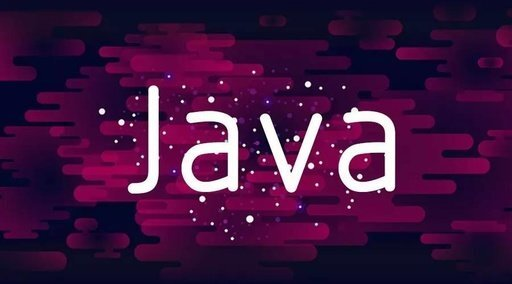Java 正则表达式

正则表达式语法规则

• [abc] 表示 a、b 或 c（简单类）

• [a-zA-Z] 表示 a 到 z 或 A 到 Z

• \d 表示 数字：[0-9]

• \D 表示 非数字

• \s 表示 空白字符（如空格、\t 制表位、\n 换行等）

• \S 表示 非空白字符（除了空白字符以外的其他都可以）

• \w 表示 单词字符：[a-zA-Z_0-9]

• \W 表示 非单词字符

• X{n} 表示 X, 恰好出现 n 次

• X{n} 表示 X, 至少 n 次

• X{n,m} 表示 X, 至少 n 次，但不超过 m 次

• 简单的 HTML

• 简单的 CSS

• 掌握 Servlet

开发工具

前端开发小编选择的是：HBuilder X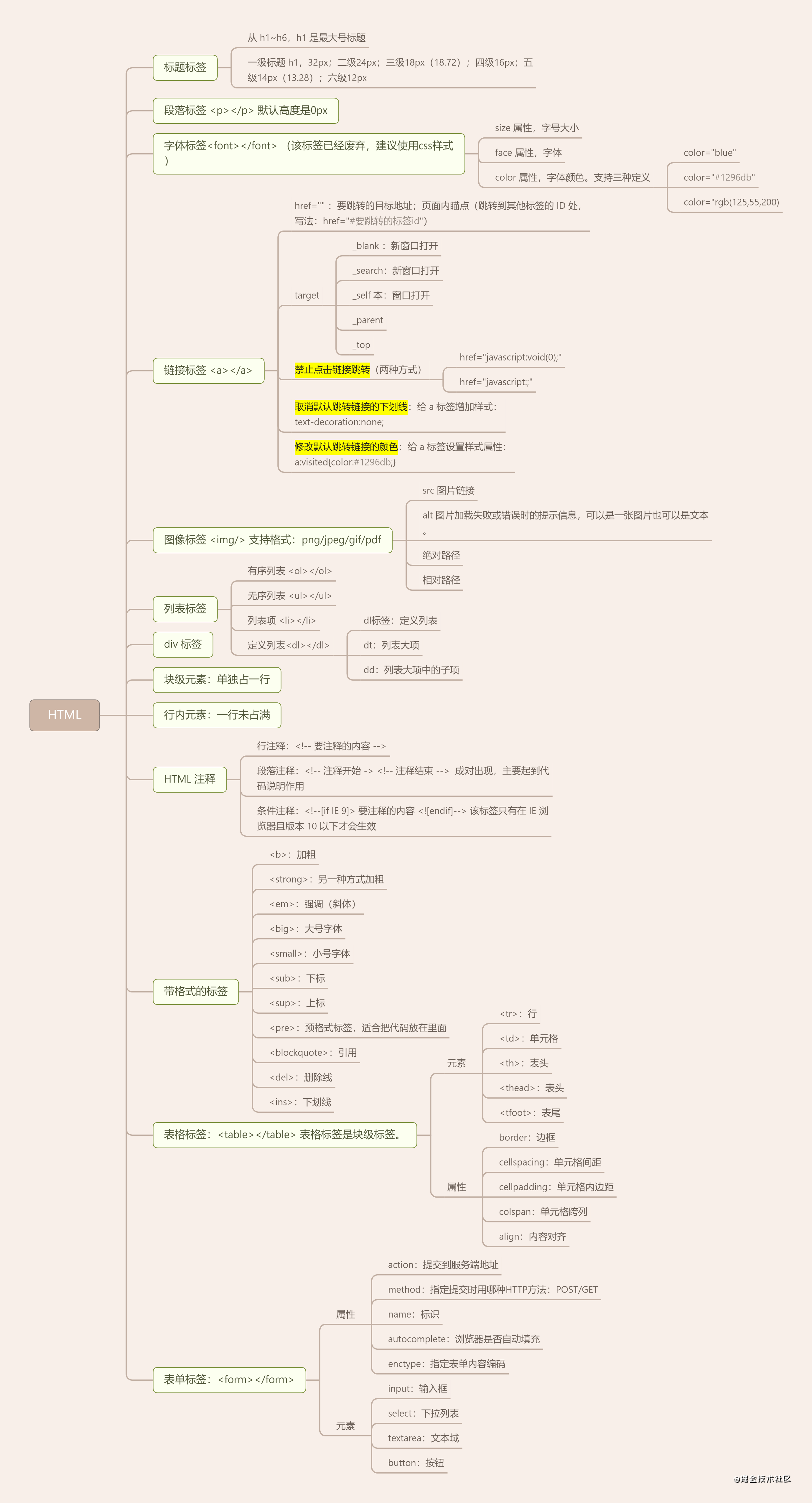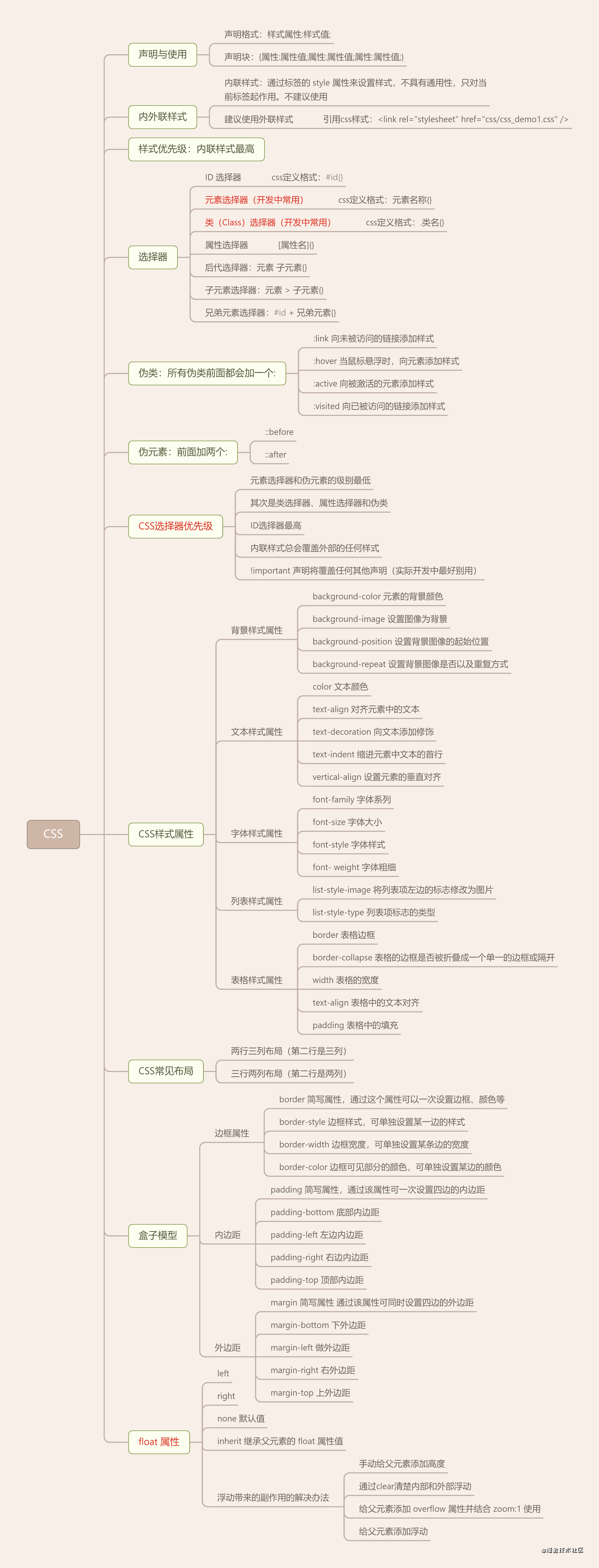Java 开发小编选择的是：IntelliJ IDEA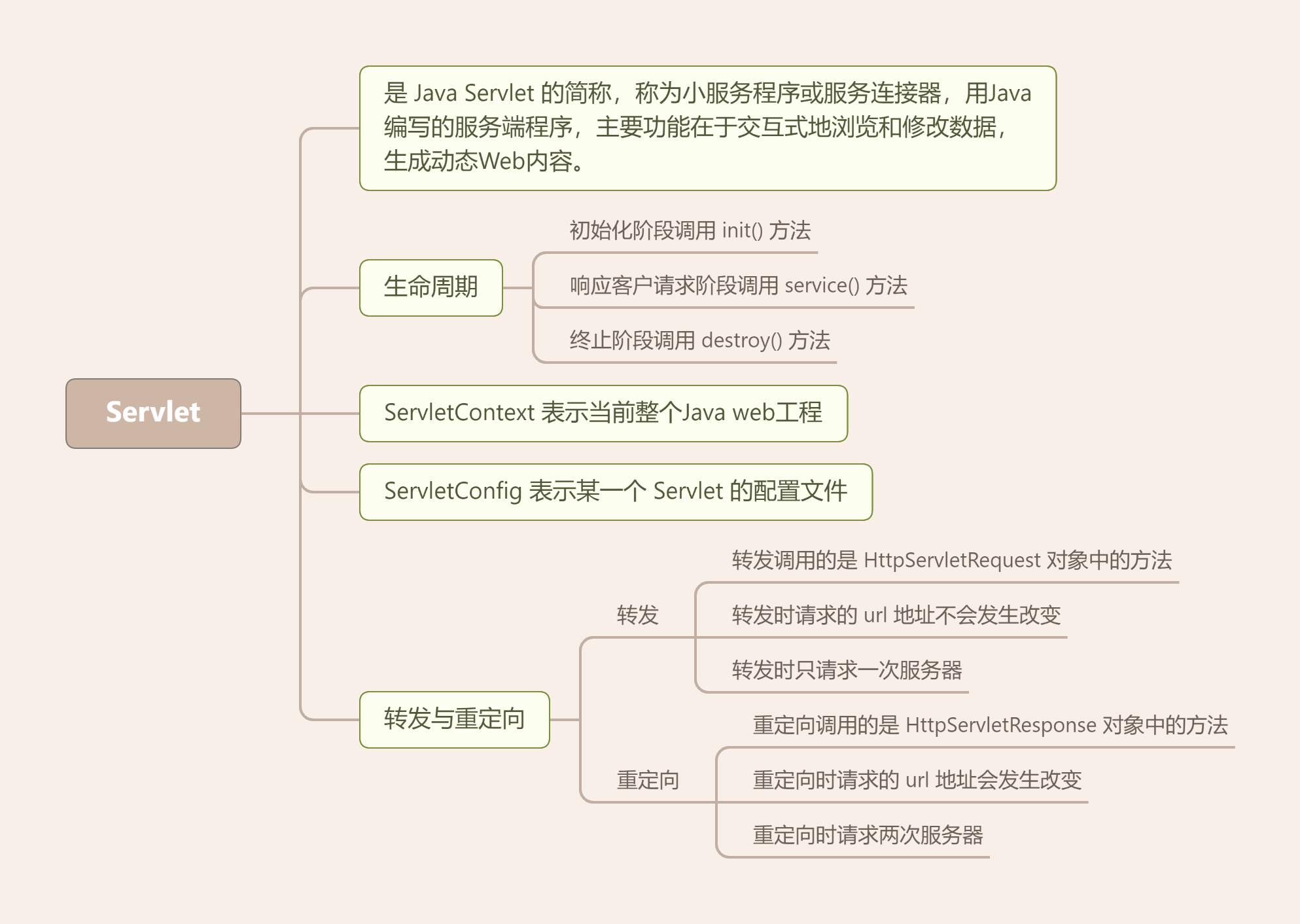Java 后端环境

• Tomcat 环境配置

• 创建 Java web 项目

• 部署 Java Web 项目

• Java Web 项目目录结构说明

• 运行 Java Web 项目

Tomcat 环境配置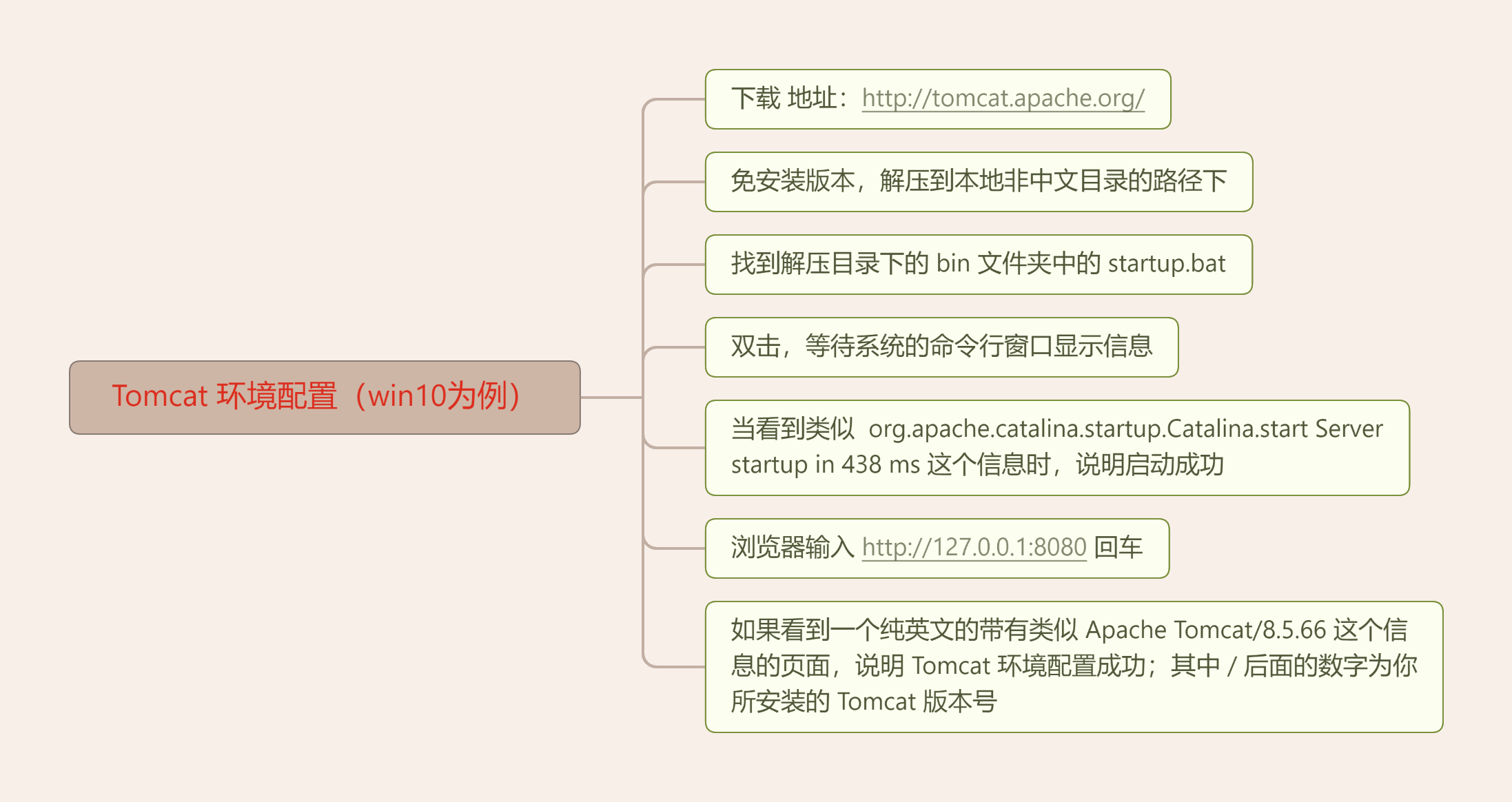创建 Java web 项目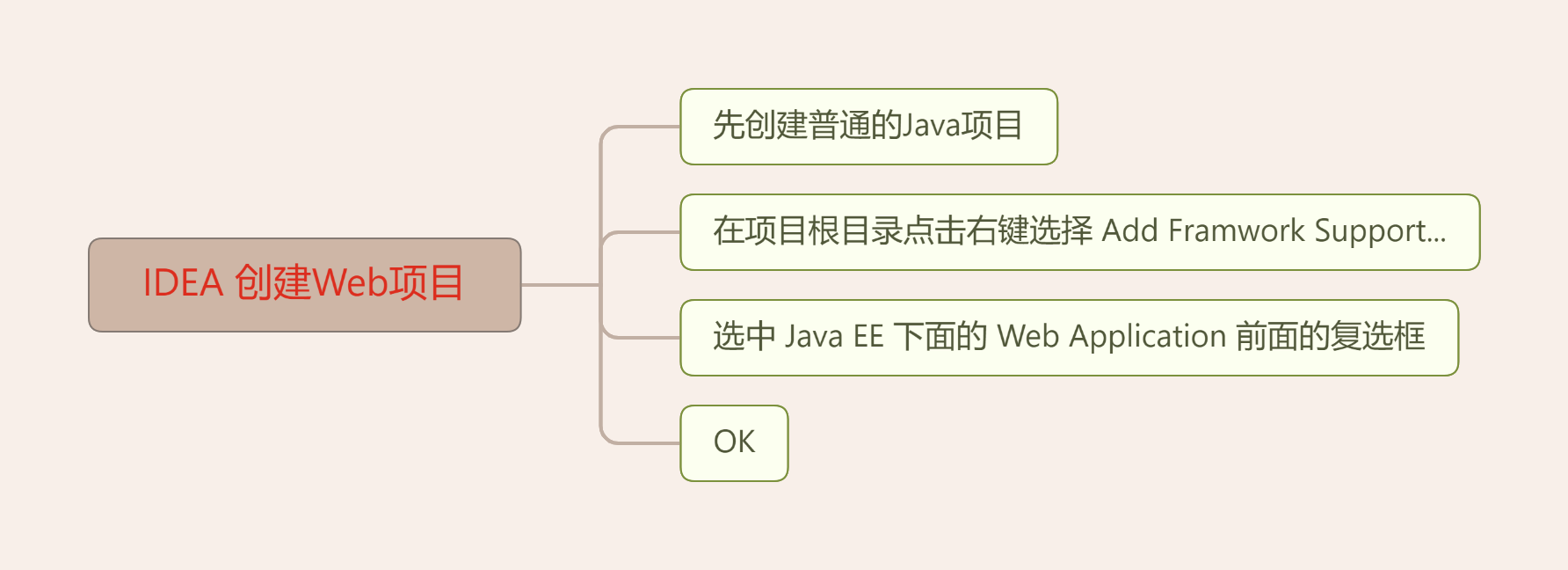部署 Java Web 项目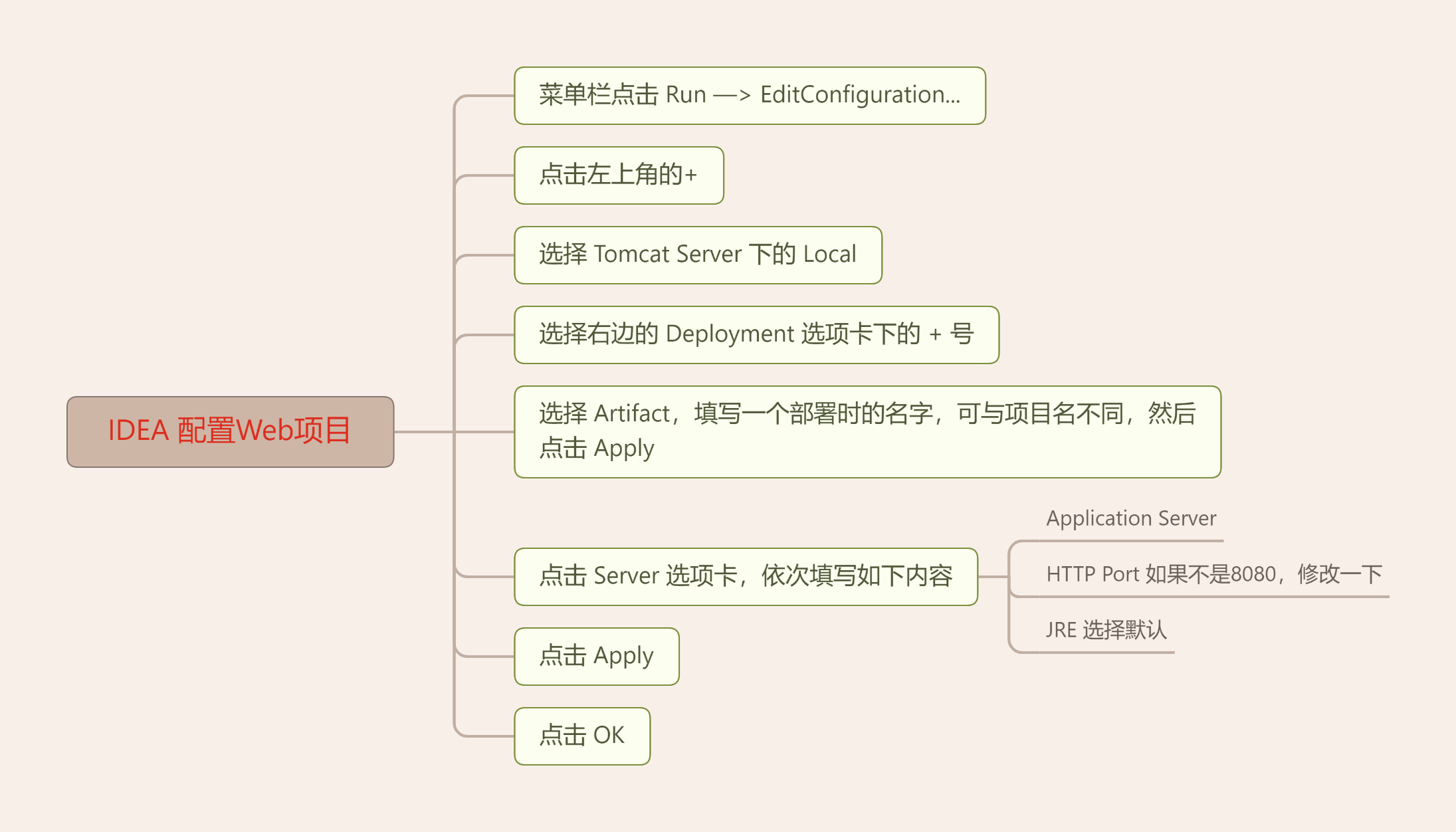总结评论